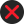19 Calculation of VAT Payable (1) Subject to sub-rule 2, the tax payable on a taxable sale is calculated by applying the rate of VAT specified in the Act to the sale price of the transaction; (2) Where the sale price is to be determined under sub-section (2) of Section 11, the VAT payable shall be calculated by the formula (T x R), where T is the consideration received for the taxable sale and R is the tax fraction. The consideration minus the VAT calculated by the above formula is the sale price; (3) The tax payable by a VAT dealer for a tax period shall be calculated by the formula, X-Y where X is a total of the VAT payable in respect of all taxable sales made by the VAT dealer during the tax period, and Y is the total input tax credit the VAT dealer is eligible to claim in the tax period under the Act. (4) Where any dealer gets himself registered for VAT under sub-section (3) of Section 17, within the time prescribed, the liability for VAT shall be from the effective date of registration,- (5) Omitted.